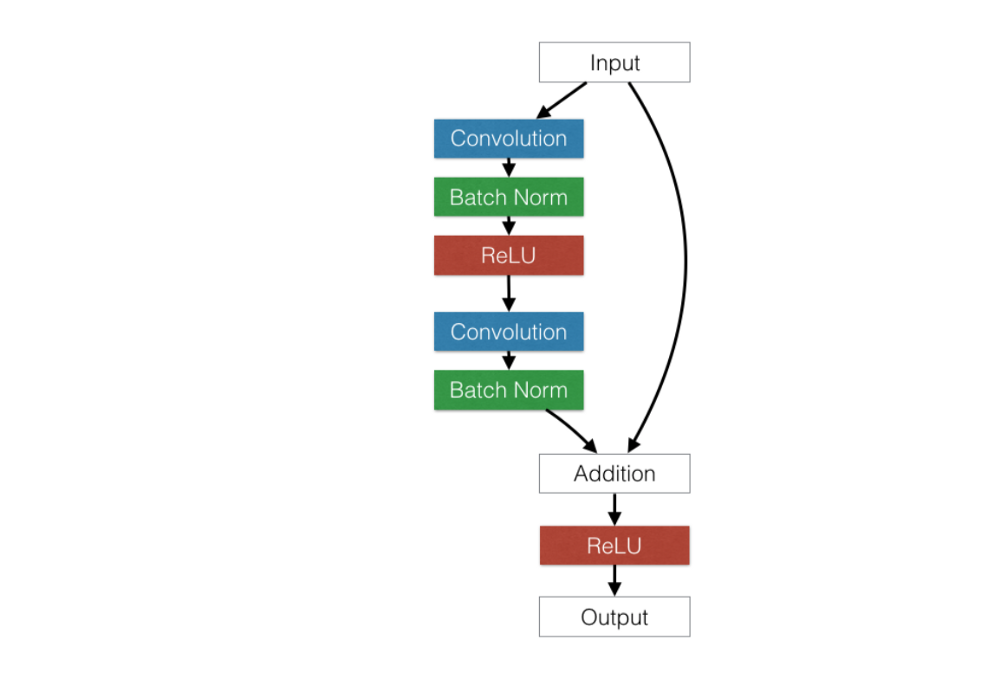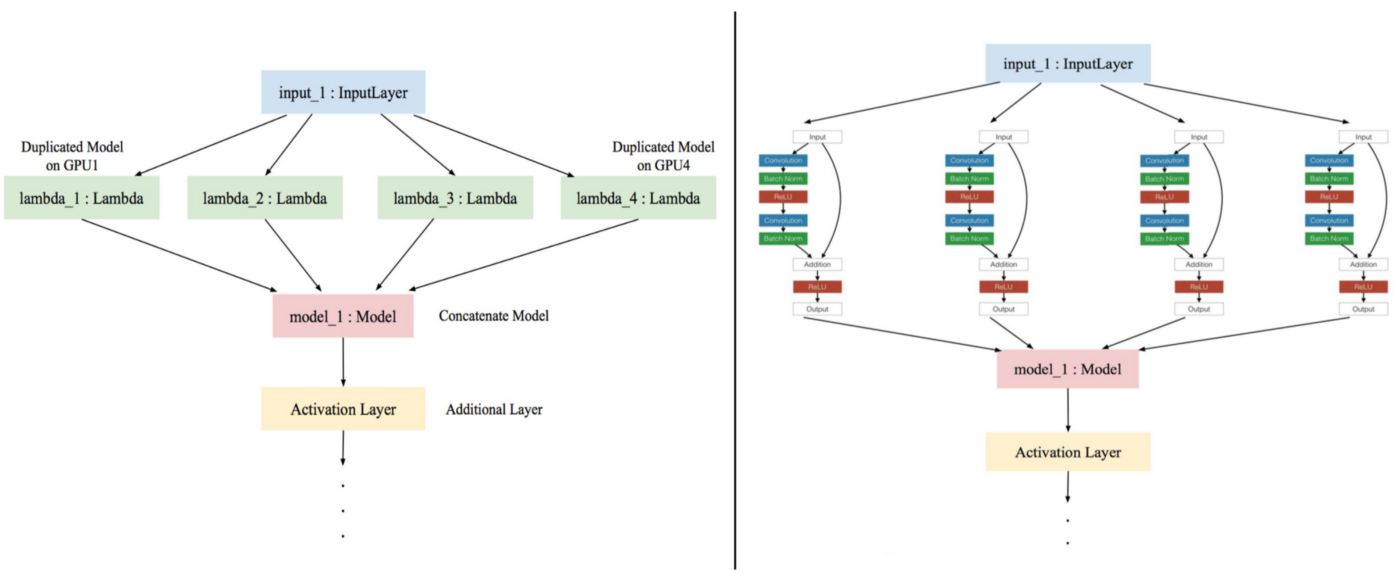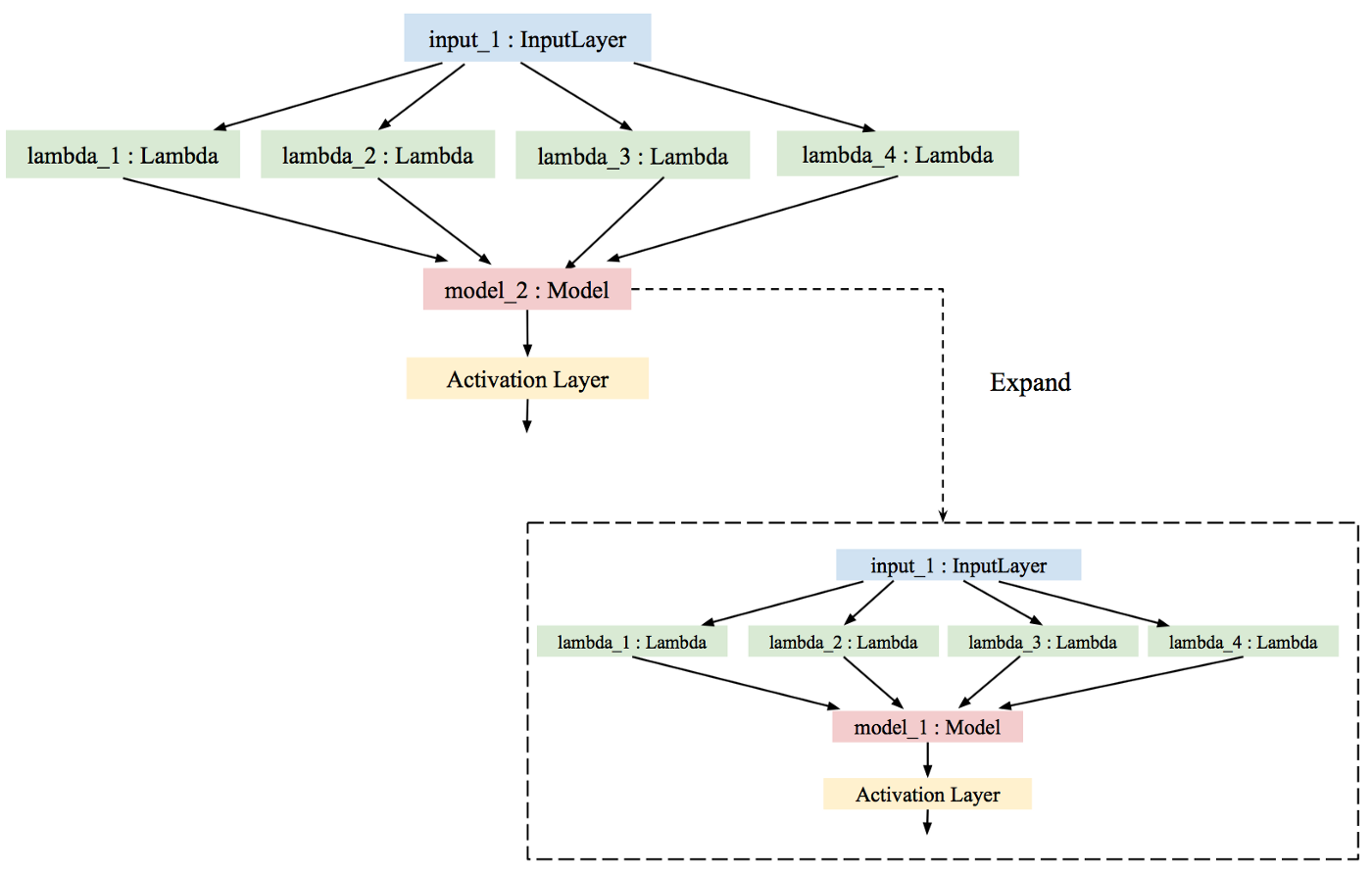## Multi-GPU Model Keras

How to

The concept of multi-GPU model on Keras divide the input’s model and the model into each GPU then use the CPU to combine the result from each GPU into one model.

#### How-To: Multi-GPU training with Keras

First, to ensure that you have Keras 2.1.4 (or greater) installed and updated in your virtual environment

• Install Keras

pip install keras pip install -- upgrade keras [in case of you’re already have keras]

• Install tensorflow gpu — to solve the problem of error ‘cudnn’, use ‘conda install’ instead of ‘pip install’

condo install tensorflow conda install tensorflow-gpu

#### Import required packages

To run the multi-GPU model on train.py

``````from keras.utils.training_utils import multi_gpu_model
from tensorflow.python.client import device_lib
import tensorflow as tf
``````

#### Check the GPUs available

``````# getting the number of GPUs
def get_available_gpus():
local_device_protos = device_lib.list_local_devices()
return [x.name for x in local_device_protos if x.device_type    == ‘GPU’]
num_gpu = len(get_available_gpus())
``````

**note that the number of gpu to run the model must be an even number.

#### How to deal with the number of GPUs

``````a = Input(shape=(32,))
b = Dense(32)(a)

# check to see if we are compiling using an odd number of gpu
# exit the program and must use the even number of gpu instance or go to the condition of one gpu.
if (num_gpu >= 2) and (num_gpu % 2 != 0):
print("Please use an even number of gpu for training!")
sys.exit()
# an even number of gpu, then run model with multi-gpu.
else if (num_gpu >= 2) and (num_gpu % 2 == 0):
#using the cpu to build the model
with tf.device('/cpu'):
model = Model(inputs=a, outputs=b)
#compile the model with gpu
multi_model = multi_gpu_model(model, gpus=num_gpu)
multi_model.compile(self, optimizer, loss=None,     metrics=None, loss_weights=None, sample_weight_mode=None, weighted_metrics=None, target_tensors=None)
# one gpu, then run as normal model
elif not (even_num_gpu or odd_num_gpu):
model = Model(inputs=a, outputs=b)
``````

To fix bug ‘TypeError: can't pickle NotImplementedType objects’ . Remind again, ensure that you have Keras 2.1.4 (or greater).

This bug causes the error when we save or load the model.

• Go to keras.utils.training_utils.py, move import tensorflow as tf as global
• Also add “import tensorflow as tf” right below def get_slice()

The structure of multi-gpu model is different from one gpu model.Assume our chosen model is ResNet as the picture on the left side. The ResNet model is wrapped into the lambda layer. The number of lambda unit depends on the number of GPU.

If you extract one lambda layer in the multi-GPU model, the structure is similar to the ordinary model that runs on one GPU.

First, use the CPU to build the baseline model, then duplicate the input’s model and the model to each GPU. Each GPU compiles their model separately then concatenates the result of each GPU into one model using the CPU.Left side : multi-GPU model. Right side : the extracted lambda layer in multi-GPU model.

If you want to load multi-GPU model. You have to use the concatenate layer to compile. So, extracting the concatenate layer in multi-GPU model (the pink one in the picture above):

``````model = models.load_model('source model path')
# Extracting the multi-gpu model.
# Extract the concatenate model layer (the pink one)
model = model.layers[-2] #or model = model.layers[-1]
then coding to compile the model as normal ...
``````

if you do not extract the concatenate layer but use the original multi-GPU model instance the result will look like thisThe model will not be loaded correctly. Further, you will notice that loading the model takes ages. This happens because each time you save the model and load it inappropriately, the concatenate layer contains another model. The second time you load the model, you repeat the process and you have three models within your model! As you load the pre-trained model, your model gets nested again and again. Eventually, loading the model could take up to hours…!

Multi-GPU training on Keras is extremely powerful, as it allows us to train, say, four times faster. However, it must be used with caution. If used incorrectly, you may run into bad consequences such as nested models, and you’re very likely won’t be able to load it to do predictions.